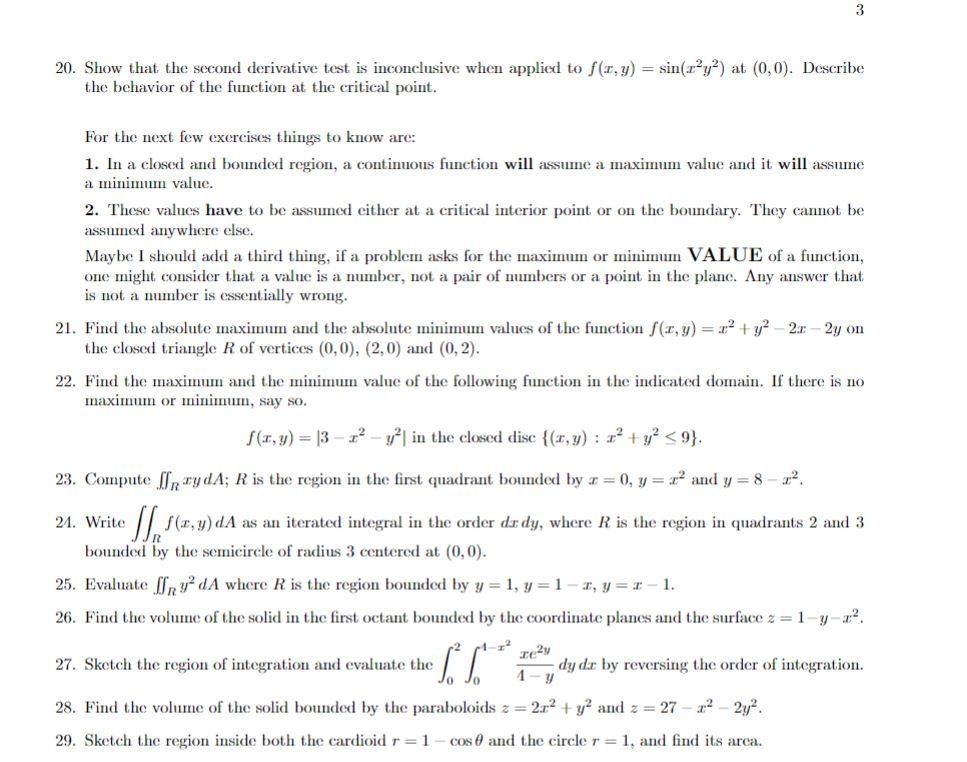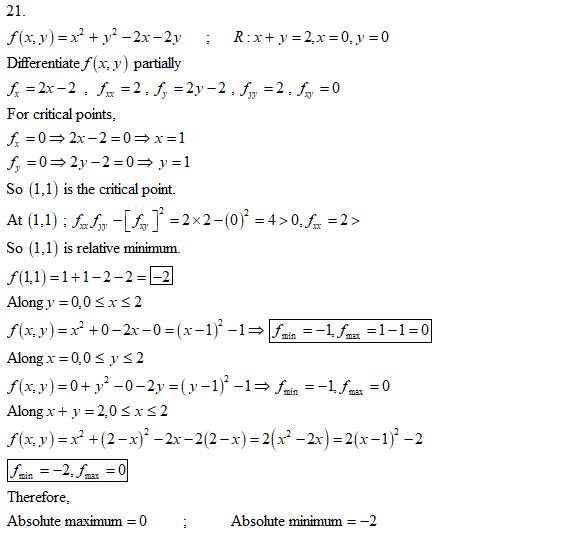Homework Help Question & Answers

20. Show that the second derivative test is inconclusive when applied to f(r, y) 2 at (0,0). Desc...20. Show that the second derivative test is inconclusive when applied to f(r, y) 2 at (0,0). Describe the behavior of the function at the critical point For the next few exercises things to know are: 1. In a closed and bounded region, a continuous function will assume a maximum value and it will assume ImIIm valuic. 2. These values have to be assumed either at a critical interior point or on the boundary. They canot be assumed anywhere else. Maybe I should add a thid thing, if a problem asks for the maxior m VALUE of a function, one might consider that a value is a number, not a pair of numbers or a point in the plane. Any answer that is not a nmber is essentially wrong 21. Find the absolutemaxiuand the absolute miu vles of theution f(x,y)y2-2r 2y on the closed triangle R of vertices (0,0), (2,0) and (0,2) 22. Find the maximu and the mium value of the following function in the indicated domain. If there is no maxIInI or immiII,SaLy so z2 y2l in the closed disc { (z, y) : z угAdd Answer of: 20. Show that the second derivative test is inconclusive when applied to f(r, y) 2 at (0,0). Desc...
More Homework Help Questions Additional questions in this topic.

• D Question 2 1 pts What are some of the implications of the Institutional Imperative Buffett desc...

Need Online Homework Help?

Get FREE EXPERT Answers
WITHIN MINUTES
Related Questions